Categories

# Atmospheric Stability

## The Hydrostatic Approximation

The vertical variation of atmospheric pressure can be deduced from hydrostatic equilibrium,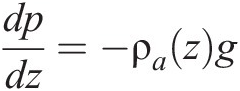(a)

which expresses that the downward gravitational force acting on a fluid parcel is balanced by an upward force exerted by the vertical pressure gradient that characterizes the fluid. Here g = 9.81 m s–2 is the acceleration of gravity, ρa [kg m–3] the mass density of air, p [Pa] the atmospheric pressure, and z [m] is the altitude above the surface, often referred to as the geometric altitude. Equation (a) assumes that the fluid parcel is in vertical equilibrium between the gravitational and pressure-gradient forces, or more broadly that any vertical acceleration of the air parcel due to buoyancy is small compared to the acceleration of gravity. This is called the hydrostatic approximation. It is a good approximation for global models, but not for small-scale models attempting to resolve strong convective motions.Because the atmosphere is thin relative to the Earth’s radius (6378 km), g can be treated as constant with altitude. From the ideal gas law, we can rewrite equation (a) as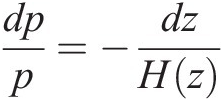(b)

where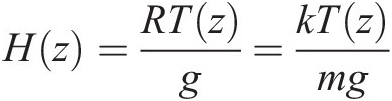(c)

is a characteristic length scale for the decrease in pressure with altitude z and is called the atmospheric scale height. Its value is 8 ± 1 km in the troposphere and stratosphere. Here

k = 1.38 × 10–23 J K–1

is Boltzmann’s constant,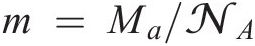is the mean molecular mass of air (28.97 × 10–3/6.022 × 1023 kg = 4.81 × 10–26 kg), and R = 287 J K–1 kg–1 is the specific gas constant for air. By integrating (b), one finds the vertical dependence of atmospheric pressure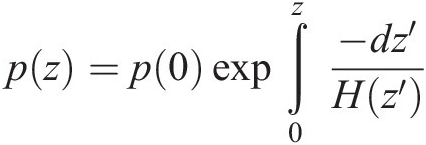(d)

where p(0) is the surface pressure. Approximating H as constant yields the simple expression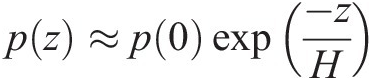(e)

which states that the air pressure decreases exponentially with altitude. Equation (e) is called the barometric law.The hydrodynamic equations of the atmosphere are often expressed by using the pressure p rather than the altitude z as the vertical coordinate. It is then convenient to define the log-pressure altitude Z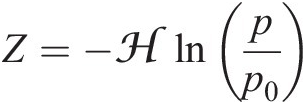(f)

as the vertical coordinate. Here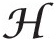is a constant “effective” scale height (specified to be 7 km) and p0 is a reference pressure (specified to be 1000 hPa). Thus Z depends solely on p. It is also convenient to introduce the geopotential Φ as the work required for raising a unit mass of air from sea level to geometric altitude z: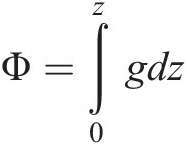(g)

where g includes dependences on altitude and latitude, the latter due to the non-sphericity of the Earth. g at Earth’s surface varies from 9.76 to 9.83 m s–2. The hydrostatic law relates Φ to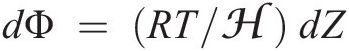.

The geopotential height is defined as Φ/go, where go = 9.81 m s–2 is a constant called the standard gravity. Movement along a surface of uniform geopotential height involves no change in potential energy, i.e., no conversion between potential and kinetic energy. Meteorological weather conditions aloft are often represented as contour maps of geopotential heights at a given pressure. As we will see in Section g, air motions tend to follow contour lines of geopotential heights, so this type of map is very useful.